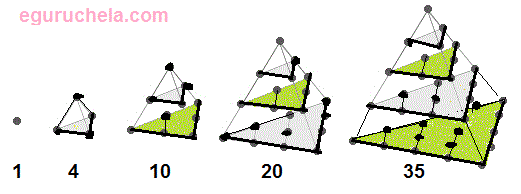# Tetrahedral Number Calculation

 Non-Negative Number (n) :

 Tetrahedral Number (Tn) =

Compute a number that can be represented by a regular geometric arrangement of equally spaced points for entered non-negative number using the following formula :

Tetrahedral Number (Tn) = 1/6n(n+1)(n+2).Tetrahedral numbers correspond to placing discrete points in the configuration of a tetrahedron (triangular base pyramid). Tetrahedral numbers are the sum of consecutive triangular numbers.

The first few tetrahedral numbers are 1, 4, 10, 20, 35, 56, 84, 120, ...